Finding the constraint equation of a circuit with a dependent voltage

• Engineering
Homework Statement:
Use the node voltage method to find v0 in the circuit shown.
Relevant Equations:
(V0-v1)/R1 + (v1-v2)/R2+…= 0
What I’m stuck on is finding the constraint equation for i on the top question . I don’t know how to find it when it’s through a voltage source and not over any resistors. (I can’t use ohm‘s law) After I find i the problem should become easy to solve. I know that v1 = 10 and v2 = 20i . The KCL for v0 is (v0-10)/10 + v0/24 + (v0-20i)/20. I could write the KCL for v1 and v2 but that doesn’t address how to find the constraint which is what I need. And when I write them and solve for my answer is way off. Something tells me I need a new approach.

Attachments

•4A7D5645-0F74-4012-AAC2-B4EB93CA2732.jpeg
55 KB · Views: 3

BvU
Homework Helper
I could write the KCL for v1 and v2
Do us a favour and write them out. There also is a KCL for the bottom node; it should read something like $$i_\Delta + {V_0\over 40\;\Omega}-20\,i_\Delta = 0$$ if I am not mistaken (not an expert here, just searching for ##n## equations with ##n## unknowns)

The KCL for v0 is (v0-10)/10 + v0/24 + (v0-20i)/20
I suppose you mean $${10\; {\sf V}- V_0\over 10\;\Omega} - {V_0\over 40\;\Omega} + {V_2-V_0\over 20\;\Omega} = 0 \qquad ?$$(considering the penciled arrows)

##\ ##

Last edited:
For v1: -i +(v1-v0)/10 + (v1 - v2)/30
For v2: I don’t know the current going through the dependent voltage source but I do know that current + (v2-v0)/20 + (v2-v1)/30.

BvU
Homework Helper
Ah, my bad: it's not a dependent current source but a dependent voltage source.
Let me call ##i_3## the current going into the bottom node (named V3...) from the right
So thus far we have \begin{align*} {V_0- V_1\over 10\;\Omega} + {V_0\over 40\;\Omega} + {V_0-V_2\over 20\;\Omega} &= 0 \tag{V0}\\ \\ -i_\Delta +{V_1-V_0\over 10\;\Omega} + {V_1 - V_2\over 30\;\Omega}&=0\tag{V1}\\ \\ i_3 + {V_2-V_0\over 20\;\Omega} + {V_2 - V_1\over 30\;\Omega}&=0\tag{V2} \\ \\ i_\Delta + {V_0\over 40\;\Omega}-i_3 &= 0 \tag{V3} \\ \\ V_1 &= 10\; {\sf V} \\ \\ V_2 &= -20\;i_\Delta \end{align*}and now I count 6 equations with only 5 unknowns -- so I must be doing something wrong, still ...

Oh, well, an opportunity to learnBy the way, the homework statement states 'use the voltage node method' and it looks as if we use the current node method so far... ?

##\ ##

Last edited:
Ah, my bad: it's not a dependent current source but a dependent voltage source.
Let me call ##i_3## the current going into the bottom node (named V3...)
So thus far we have \begin{align*} {V_0- V_1\over 10\;\Omega} + {V_0\over 40\;\Omega} + {V_0-V_2\over 20\;\Omega} &= 0 \tag{V0}\\ \\ -i_\Delta +{V_1-V_0\over 10\;\Omega} + {V_1 - V_2\over 30\;\Omega}&=0\tag{V1}\\ \\ i_3 + {V_2-V_0\over 20\;\Omega} + {V_2 - V_1\over 30\;\Omega}&=0\tag{V2} \\ \\ i_\Delta + {V_0\over 40\;\Omega}-i_3 &= 0 \tag{V3} \\ \\ V_1 &= 10\; {\sf V} \\ \\ V_2 &= -20\;i_\Delta \end{align*}and now I count 6 equations with only 5 unknowns -- so I must be doing something wrong, still ...

Oh, well, an opportunity to learnBy the way, the homework statement states 'use the voltage node method' and it looks as if we use the current node method so far... ?

##\ ##
I'm in school currently, but when I have some down time I'll try setting the node labeled v2 as the ground. I think this might help out because the 60 V source would be in-between the current ground node and v1, which would make a supernode and give a constraint equation for idelta in terms of v1 and the current ground.

DaveE
Gold Member
By the way, the homework statement states 'use the voltage node method' and it looks as if we use the current node method so far... ?
OK, I had to look that up. I also wasn't familiar with the name. Khan Academy says "voltage node method" is what I call KCL.

Last edited:
BvU
Homework Helper
I had a fun day learning the quirks of Qucs (quite universal circuit simulator).
Almost converted me to Linux, but that curve I don't think I can handle, so I'll have to live with a buggy windows version.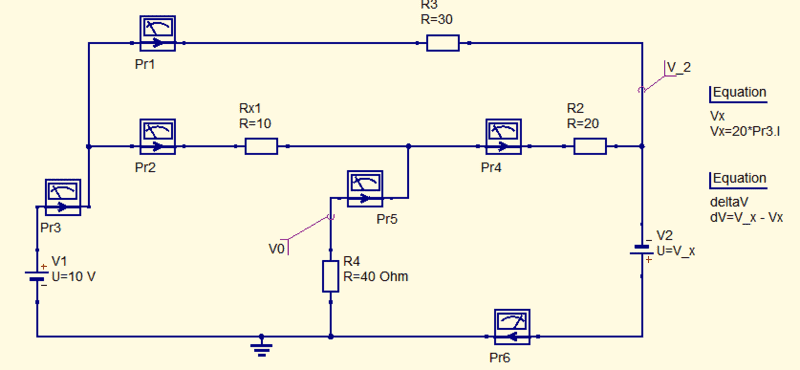(You wouldn't believe where you have to go to turn off the grid points in the circuit diagram)

Can't make it come up with a sensible answer when I use ##\ 20\ i_\Delta\ ## for the dependent voltage (gets 15.2 V but claims V2 = 0 at the same time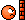).

However, when I let step through a range of voltages, a solution emerges. Suppose I can't spoil it under PF rulesbut at least I can use it to see if the equations in the list are satisfied. Tomorrow (1 AM here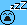)...

Hint: do the exercise with ##\infty## for the 40 ##\Omega## resistor ...piece of cake !

##\ ##

DaveE
Gold Member
I had a fun day learning the quirks of Qucs (quite universal circuit simulator).
Have you tried LTSpice? What does Qucs in comparison have that makes it worth my time to investigate?

BvU
Homework Helper
Didn't know either this morning, so just tried one. ( see post #2)

•DaveE
DaveE
Gold Member
Didn't know either this morning, so just tried one. ( see post #2)
You might try LTSpice then. It's the most popular free simulator. Of course it will be annoying learning a new system. It always is.

BvU
Homework Helper
Here is an LTspice .asc file
Very nice. Yet another wrench to get used to in my toolkit. First impression is better than Qucs (but I am slightly prejudiced - Analog Devices does that for me).

However, my intuition doesn't help me here: how is this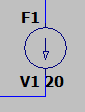a dependent voltage source delivering ##20 * i_\Delta ## Volts ?

@johnsmith7565 : what do you find with my suggestive hint in #7 at the end?

##\ ##

Baluncore
2021 Award
... a dependent voltage source delivering 20 * iΔ Volts ?
Look up "F" in the help and you will see that it is a primitive current controlled current source.
The current flowing through voltage source V1 is multiplied by 20.

BvU
Homework Helper
Can't find a simple current controlled voltage source, but a fixed voltage source with the value as found in #7 does the trick and gets the exact same result. Thanks!

Now on to doing things by hand and analytically ..counting and checking out eqns in #4

##\ ##

Baluncore
2021 Award
Can't find a simple current controlled voltage source, ...
E. Voltage Dependent Voltage Source.
F. Current Dependent Current Source.
G. Voltage Dependent Current Source.
H. Current Dependent Voltage Source.

There is now a more flexible general element.
B. Arbitrary Behavioral Voltage or Current Sources.

•BvU
BvU
Homework Helper
Boy, the stupidities one can come up with ! in #4
$$i_\Delta + {V_0\over 40\;\Omega}-i_3 = 0 \tag{V3}$$should of course be
$$i_\Delta - {V_0\over 40\;\Omega}-i_3 = 0 \tag{V3}$$(all currents going into bottom node V3).

And then the equations are not independent (## V0+V1+V2+V3\Rightarrow 0=0\ ##) so we are left with 5 eqns with 5 unks. I will be able to sleep again.

Oops, forgot to also solve the set ...----

E. Voltage Dependent Voltage Source.
...

Excavated that F2 allows inserting some things, but never guessed what h stands for.
Now embark on an expedition to enforce H1.value = 20 * V1.I ? (in my case -20, because flipping a symbol is too expert for meas well..

How come every different program is as if learning a different language on a different planet?

##\ ##

Baluncore
2021 Award
... because flipping a symbol is too expert for me ...
Control R will Rotate selected symbol clockwise.
Control E will flip selected symbol horizontal.

Last edited:
•BvU
BvU
Homework Helper
You are too indulgent. I'm just moping: why can't the program tell met that ? Searching help for flip or rotate yields no help whatsoever. I suppose being patient and working through yet another 'getting started' should do the trickBaluncore
2021 Award
You are too indulgent. I'm just moping: why can't the program tell met that ?
The LTspice learning curve is exciting, as it has some overhangs.
There may still be a hint if you look in the edit pull down menu.
If you cannot see a function, ask here so others can benefit.

Help - Contents - Schematic capture - Keyboard shortcuts - - -
Help - Search - “rotate” - List topics - Schematic editing - - -

Last edited:
•BvU
Very nice. Yet another wrench to get used to in my toolkit. First impression is better than Qucs (but I am slightly prejudiced - Analog Devices does that for me).

However, my intuition doesn't help me here: how is this
a dependent voltage source delivering ##20 * i_\Delta ## Volts ?

@johnsmith7565 : what do you find with my suggestive hint in #7 at the end?

##\ ##
Sorry for not responding earlier.

Trying to make the other node a ground node went no where.

To be honest I am a little bit confused... why would I plug in infinity for the 40 ohm resistor? How do I even do that? This problem seems very difficult for a basic text on node voltages! It seems like there is some simple trick to make this problem easier to solve...

DaveE
Gold Member
You are too indulgent. I'm just moping: why can't the program tell met that ? Searching help for flip or rotate yields no help whatsoever. I suppose being patient and working through yet another 'getting started' should do the trickYes, it's a pain. I've never liked the schematic capture part of LTSpice, but it does work well.
There is some good stuff online about how to use it. This one looked good to me. http://www.simonbramble.co.uk/lt_spice/ltspice_lt_spice.htm

•BvU
I taught myself the loop current method today, and I've just solved this circuit using the loop-current method. IMO mush easier and straightforward with the circuit using the loop-current method.

•BvU
BvU
Homework Helper
Trying to make the other node a ground node went no where.
Not surprised.
To be honest I am a little bit confused... why would I plug in infinity for the 40 ohm resistor? How do I even do that?
Because that makes it a very simple circuit. And the results are useful when estimating which way the actual solution should go. Cryptic ?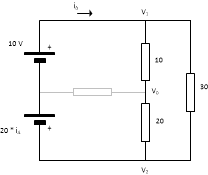Try to solve this one (so without the 40 Ohm) for V0This problem seems very difficult for a basic text on node voltages! It seems like there is some simple trick to make this problem easier to solve...
I agree with you. But I would be surprised if there was a trick. I do suppose the set of equations can be solved, but it's work and I am thoroughly lazy.

The Electrician
Gold Member
I taught myself the loop current method today, and I've just solved this circuit using the loop-current method. IMO mush easier and straightforward with the circuit using the loop-current method.
What result did you get?

What result did you get?
24 V for v0.